# 卷积神经网络基础之百度飞浆课程笔记

### 卷积（Convolution）

#### 卷积计算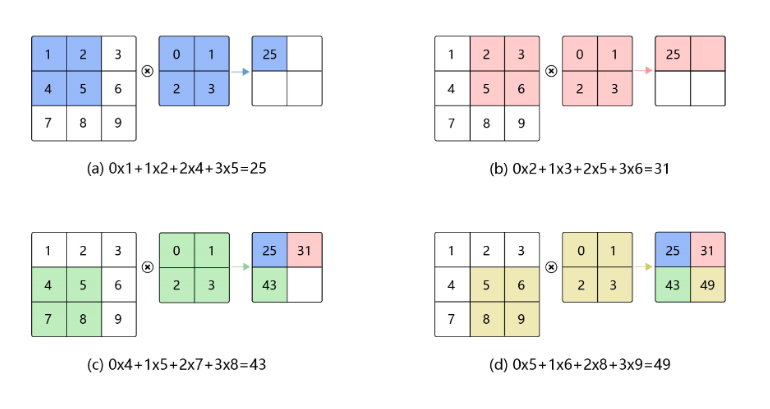\begin{aligned} H_{\text {out}} &=H-k_{h}+1 \\ W_{\text {out}} &=W-k_{w}+1 \end{aligned}

$H$表示输入图片的高度，$W$表示输入图片的宽度，$k_{h}$ 表示卷积核的高度，$k_{w}$表示卷积核的宽度，$1$ 表示偏置项，$H_{out}$表示输出尺寸的高，$W_{out}$表示输出尺寸的宽。（尺寸计算一定要会，很基础也很重要）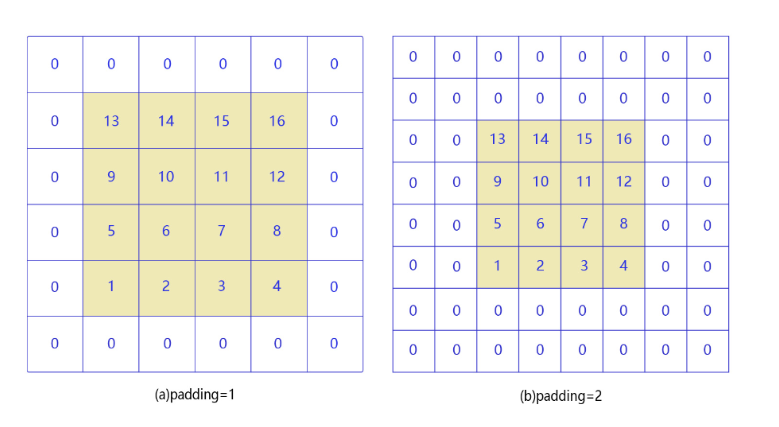$\begin{array}{l} H_{\text {out}}=H+p_{h 1}+p_{h 2}-k_{h}+1 \\ W_{\text {out}}=W+p_{w 1}+p_{\text {w} 2}-k_{w}+1 \end{array}$

\begin{aligned} H_{\text {out}} &=H+2 p_{h}-k_{h}+1 \\ W_{\text {out}} &=W+2 p_{w}-k_{w}+1 \end{aligned}

#### 步幅（stride）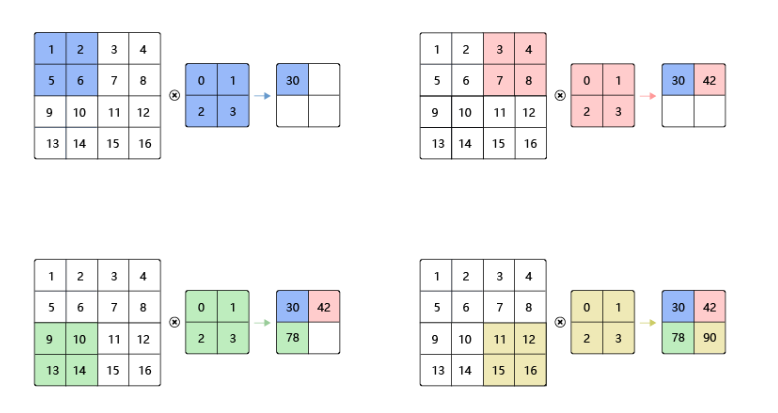\begin{aligned} H_{\text {out}} &=\frac{H+2 p_{h}-k_{h}}{s_{h}}+1 \\ W_{\text {out}} &=\frac{W+2 p_{w}-k_{w}}{s_{w}}+1 \end{aligned}

#### 多通道操作

• 多输入通道场景

假设图片的通道数为$C_{in}$，输入数据的形状为$C_{in}\times H_{in}\times W_{in}$ ，则计算过程如下图所示：

1. 对每一个通道分别设计一个2维数组卷积核，所以卷积核数组的形状为$C_{in}\times k_{h}\times k_w$
2. 对任意一个通道，分别用对应通道的卷积核进行卷积运算。
3. 将这$C_{in}$ 个通道各自的卷积计算结果相加，得到一个形状为$H_{out}\times W_{out}$ 的二维数组。
4. 其中$H_{out}\times W_{out}$ 的大小依据单通道的公式计算即可。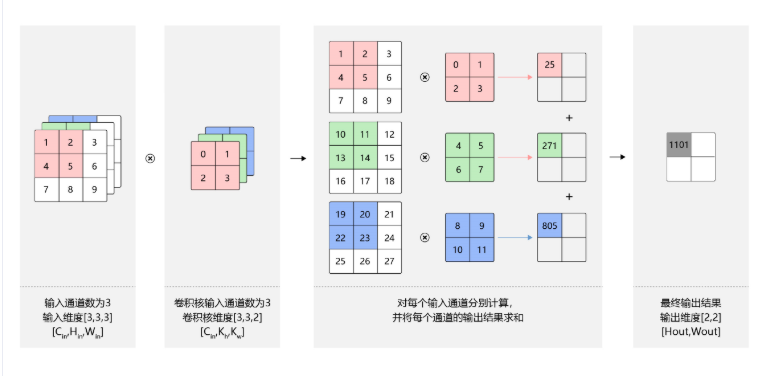• 多输出通道场景

一般来说，卷积操作的输出特征图也具有多通道$C_{out}$ ，这时我们需要设计$C_{out}$ 个维度为$C_{in}\times k_h\times k_w$ 的卷积核，这里可能不太好理解，不过只要多一点点思考就可以想明白了，其实只是在维度上增加了而已，不过实际中通常我们将多通道输出个数叫做卷积核个数，因为四维空间较为抽象，卷积核数组的最终维度为$C_{out}\times C_{in}\times k_h\times k_w$ ，如下图：

1. 对任一输出通道$c_{o u t} \in\left[0, C_{o u t}\right)$ ，都是使用上面描述的$C_{in}\times k_h\times k_w$ 卷积核对图像做卷积得到的。
2. $C_{out}$个形状为$H_{out} \times W_{out}$ 二维数组拼在一起，就得到$C_{out}\times H_{out}\times W_{out}$ 形状的多通道输出。
3. 对这个的理解，需要一步步的，首先掌握单通道的卷积尺寸计算，然后掌握多输入通道场景的计算，再然后就可以很好理解多输出通道场景的计算了。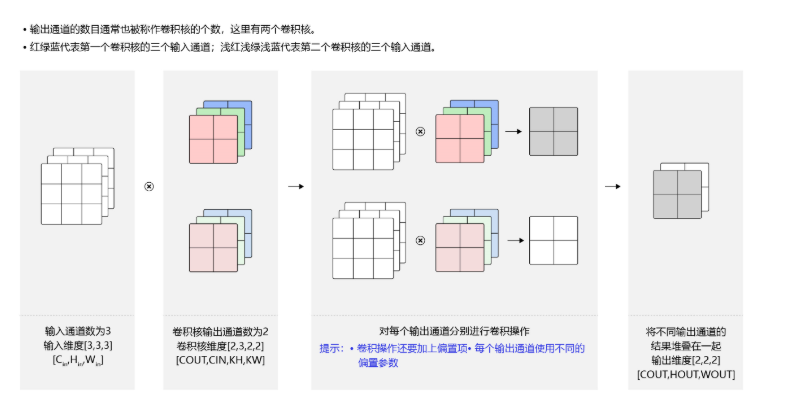• 批量操作

卷积神经网络中，通常进行多样本一起训练，即输入数据的维度为$N\times C_{in}\times H_{in}\times W_{in}$ 。由于会对每张图片使用同样的卷积核进行卷积操作，所以卷积核的计算和上述多输出通道一样，卷积核的维度仍然是$C_{out}\times C_{in}\times k_h\times k_w$ ，输出维度为$N\times C_{out}\times H_{out}\times W_{out}$ ,如下图所示：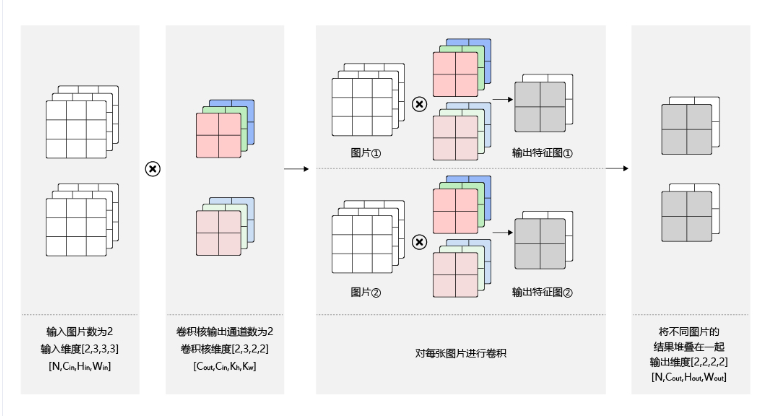### 池化（Pooling）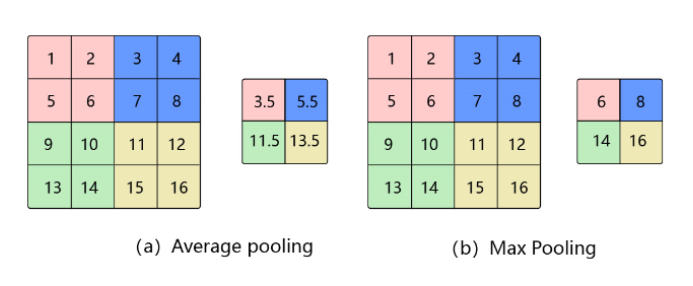\begin{aligned} H_{\text {out}} &=\frac{H+p_{h 1}+p_{h 2}-k_{h}}{s_{h}}+1 \\ W_{\text {out}} &=\frac{W+p_{w 1}+p_{w 2}-k_{w}}{s_{w}}+1 \end{aligned}

### 批归一化

#### 批归一化公式计算过程

1. 计算mini-batch内样本的均值

2. 计算mini-batch内样本的方差

3. 计算标准化之后的输出

4. 对标准化的输出进行平移和缩放

如果强行限制输出层的分布是标准化的，可能会导致模型某些特征模式的丢失，所以会紧接着需要进行平移和缩放

#### 批归一化实践技巧

1. 解决方案：训练时计算在整个数据集上的均值和方差，并将结果保存，预测时不计算样本内均值和方差，而是使用训练时保存的值

2. 计算方法：在训练过程中通过滚动平均的方式，计算在整个数据集上的均值和方差并保存，公式如下：

$\begin{array}{l} \text { saved } _{-}\mu_{B} \leftarrow \text { saved }_{-} \mu_{B} \times 0.9+\mu_{B} \times(1-0.9) \\ \text { saved }_{-} \sigma_{B}^{2} \leftarrow \text { saved_ } \sigma_{B}^{2} \times 0.9+\sigma_{B}^{2} \times(1-0.9) \end{array}$

$saved_{-}\mu_{B}$ 表示训练过程中前面所有轮次的滚动的均值；

${ saved }_{-} \sigma_{B}^{2}$ 表示训练过程中前面所有轮次的滚动的方差；

0.9表示滚动平均的动量系数（momentum)；

$\mu_{B}$ 表示本轮batch数据的均值；

$\sigma_{B}^{2}$ 表示本轮batch数据的方差；

按照上述公式不断迭代，直到训练过程结束，最后得到的均值和方差保存下来，作为预测数据归一化的均值和方差。

### 丢弃（Dropout）

#### 简介

Dropout是一种抑制过拟合的方法

#### 方法

1. 训练阶段：每次随机的删除一部分神经元，不向前传播所携带的信息，相当于每次都是让不同的模型在学习
2. 测试阶段：向前传播所有神经元的信息，相当于让不同的模型一起工作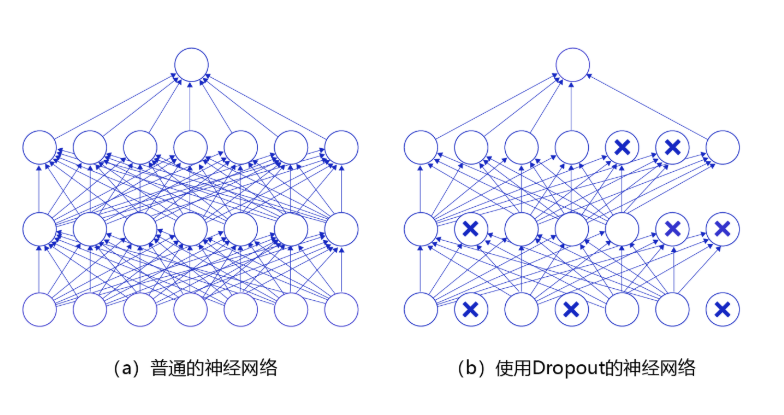### 参考资料

posted @ 2020-08-20 14:11  N.S.A  阅读(357)  评论(0编辑  收藏